# Numerical simulation of two-dimensional fluid flow problem using truly meshfree method

Numerical simulation of two-dimensional fluid flow problem using truly meshfree method

Rajul GargHarishchandra Thakur Brajesh Tripathi

School of Engineering, Gautam Buddha University, Greater Noida 201308, U.P., India

Rajasthan Technical University, Kota, Rajasthan 324009, India

Corresponding Author Email:
garg.rajul2010@gmail.com
Page:
357-364
|
DOI:
https://doi.org/10.18280/mmep.050412
23 July 2018
|
Accepted:
28 August 2018
|
Published:
31 December 2018
| Citation

OPEN ACCESS

Abstract:

The solution of two-dimensional steady and transient fluid flow problem by the truly meshless local Petrov-Galerkin (MLPG) method has been addressed in the present article. The unknown function of velocity u(x) is approximated by moving least square approximant uh(x). The essential boundary condition is imposed both by the direct and penalty function methods. Fourth order spline weight function, monomial basis function and a set of non-constant coefficients are used to construct the approximants. The two-level q method is employed for temporal discretization. The results obtained by the MLPG method are compared with the analytical solution and also with the benchmark method results and found to be in the excellent agreement.

Keywords:

steady-state analysis, transient analysis, incompressible fluid flow, direct method of interpolation, penalty function method, meshless local petrov-galerkin method

1. Introduction

Finite element method (FEM) has been established as a powerful numerical technique to address the complex problems of different engineering domains; still it is encountered with certain limitations including the mesh creation, which is a costly and time consuming task than the assembly and solution of the FE equations. Also, there are certain class of problems for which the FEM is not a suitable technique to address. To overcome these problems, a large number of meshless methods have been evolved in the recent years. Some of these methods are: smoothed particle hydrodynamics (SPH) method [1-3], diffuse element method , local boundary integral equation (LBIE) method [5-6], element free Galerkin (EFG) method [7-8], finite point method (FPM) , MLPG method [10-12], point interpolation method (PIM) , radial point interpolation method (RPIM) [14-16] and meshfree weak-strong form (MWSF) methods , etc.

However, among all the meshfree methods, the MLPG method has become quite popular due to its successful acceptability in various fields of engineering [18-23]. In this method the domain discretization originates from a weak form over a local sub-domain of arbitrary shape which is located completely inside the global domain. It constructs the global stiffness matrix through the integration over local sub-domains, naturally. Integration over local domains removes the need of any background mesh over entire domain. Thus, the proposed method does not need any mesh either at the stage of interpolation or at the stage of integration; hence, it can appropriately be called a truly meshfree method.

The MLPG method works on Petrov-Galerkin formulation i.e. it picks up test and trial functions from different function spaces. This feature provides the flexibility to formulate the discretization method in different ways. However, there is a strong base of available literature [24-31] that proves that the MLPG method is one of the promising methods to solve complex engineering problems including fluid flow problems efficiently and effectively.

In the present article, the MLPG method is used to solve the steady and transient fluid flow problems in two-dimensional space. Direct method of interpolation (DM) and penalty function methods (PM)  are used to enforce the essential boundary conditions (EBC). C++ codes have been developed to model the problem. The results obtained by the MLPG method are compared with that of the analytical and benchmark solutions.

2. The Mlpg Method

The MLPG method utilizes the MLS scheme , which consists of three components: a completely monomial basis function, a set of coefficients that depends on node position and a weight function associated with each node. The unknown function u(x) is approximated by MLS approximant uh(x) as,

${{u}^{h}}(\mathbf{x})=\sum\limits_{j=1}^{m}{{{p}_{j}}(\mathbf{x}){{a}_{j}}(\mathbf{x})}\equiv {{\mathbf{p}}^{T}}(\mathbf{x})\mathbf{a}(\mathbf{x})$  (1)

where pT(x) = (p1 (x), p2 (x),……, pm (x)) is a complete monomial basis. The coefficient vector a(x) is determined by minimizing a weighted discrete L2norm defined as,

$J=\sum\limits_{i=1}^{n}{w}(\mathbf{x},{{\mathbf{x}}_{i}}){{[{{u}^{h}}(\mathbf{x})-u({{\mathbf{x}}_{i}})]}^{2}}=\sum\limits_{i=1}^{n}{w}(\mathbf{x},{{\mathbf{x}}_{i}}){{[{{\mathbf{p}}^{\mathbf{T}}}\mathbf{(}{{\mathbf{x}}_{\mathbf{i}}}\mathbf{)a(x)}-u({{\mathbf{x}}_{i}})]}^{2}}$  (2)

where w(x, xi) is a weight function, u(xi) = uiis the nodal parameter of the field variable at node xi and n is the number of nodes in the support domain of x for which the weight function w(x,xi)≠0. The stationarity of J with respect to a(x) results in the following linear system:

$\mathbf{A(x)a(x)=B(x)u}$ (3)

The above equation can be written as,

$\mathbf{a}(\mathbf{x})={{\mathbf{A}}^{-1}}\mathbf{(x)B(x)u}$ (4)

where matrices A(x) and B(x) are defined as,

$\mathbf{A(x)}=\sum\limits_{i=1}^{n}{w}(\mathbf{x},{{\mathbf{x}}_{i}})\mathbf{p(}{{\mathbf{x}}_{\mathbf{i}}}\mathbf{)}{{\mathbf{p}}^{\mathbf{T}}}\mathbf{(}{{\mathbf{x}}_{\mathbf{i}}}\mathbf{)}$ (5)

\begin{align} & \mathbf{B(x)}=[w(\mathbf{x},{{\mathbf{x}}_{1}})\mathbf{p(}{{\mathbf{x}}_{1}}\mathbf{)},w(\mathbf{x},{{\mathbf{x}}_{2}})\mathbf{p(}{{\mathbf{x}}_{2}}\mathbf{)},... \\ & ....,w(\mathbf{x},{{\mathbf{x}}_{n}})\mathbf{p(}{{\mathbf{x}}_{n}}\mathbf{)}] \\\end{align} (6)

Substituting a(x) in Eqn. (1), the MLS approximant is obtained as,

${{u}^{h}}(\mathbf{x})=\sum\limits_{i=1}^{n}{{{\Phi }_{i}}{{u}_{i}}}=\mathbf{\Phi (x)}u$ (7)

The choice of weight function affects the resulting approximation uh(x). The weight function w(x, xi) is non-zero over a small domain in the neighbourhood of node xi. Therefore, the selection of appropriate weight function is essential in the MLPG method. The fourth order spline weight function  is used in the present analysis and can be written as,

$w(\mathbf{x},{{\mathbf{x}}_{\mathbf{i}}})=\left\{ \begin{matrix} 1-6{{d}^{2}}+8{{d}^{3}}-3{{d}^{4}} & \text{if }0\le d\le 1 \\ 0 & \text{if }d>1 \\ \end{matrix} \right.$ (8)

where $d_{i}=\left\|x-x_{i}\right\|$, i.e. the Euclidean distance from node xi to point x.

MLS shape functions do not possess Kronecker delta function property; hence, imposition of EBC is not an easy task.

3. Discretization of Governing Equations

An incompressible fluid flowing through a long and uniform duct is considered in Fig. 1.

## 1.jpgFigure 1. Model cross-section of the fluid flowing through a duct

The momentum equation for incompressible fluid flowing through a long and uniform duct is given as,

$\mu (\frac{{{\partial }^{2}}u}{\partial {{x}^{2}}}+\frac{{{\partial }^{2}}u}{\partial {{y}^{2}}})-\frac{\partial p}{\partial z}=\rho \frac{\partial u}{\partial t}$ (9)

The initial and essential boundary conditions are,

\left. \begin{align} & at\text{ }t=\text{ }0,u={{u}_{ini}} \\ & at\text{ }{{\Gamma }_{1}},\text{ }{{u}_{top\text{ }surface}}=\text{ }{{u}_{{{\Gamma }_{1}}}}=\text{ }0 \\ & at\text{ }{{\Gamma }_{2}},\text{ }{{u}_{left\text{ }surface}}=\text{ }{{u}_{{{\Gamma }_{2}}}}=\text{ }0 \\ & at\text{ }{{\Gamma }_{3}},\text{ }{{u}_{bottom\text{ }surface}}=\text{ }{{u}_{{{\Gamma }_{3}}}}=\text{ }0 \\ & at\text{ }{{\Gamma }_{4}},\text{ }{{u}_{right\text{ }surface}}=\text{ }{{u}_{{{\Gamma }_{4}}}}=\text{ }0 \\\end{align} \right\} (10)

The weighted residual formation for Eqn. (9) in local domain ΩQ can be expressed as,

$\int\limits_{{{\Omega }_{Q}}}{v\left\{ \mu (\frac{{{\partial }^{2}}u}{\partial {{x}^{2}}}+\frac{{{\partial }^{2}}u}{\partial {{y}^{2}}})-\frac{\partial p}{\partial z}-\rho \frac{\partial u}{\partial t} \right\}}d\Omega =0$ (11)

The weak form of the Eqn. (11) is obtained as,

$\int_{\Gamma }{v}\mu (\frac{\partial u}{\partial x}+\frac{\partial u}{\partial y})d\Omega -\int\limits_{{{\Omega }_{Q}}}{\left\{ \mu (\frac{\partial u}{\partial x}\frac{\partial v}{\partial x}+\frac{\partial u}{\partial y}\frac{\partial v}{\partial y}) \right\}}d\Omega -\int\limits_{{{\Omega }_{Q}}}{\left\{ v\frac{\partial p}{\partial z} \right\}}d\Omega -\int\limits_{{{\Omega }_{Q}}}{\left\{ \rho v\frac{\partial u}{\partial t} \right\}}d\Omega +\int_{\Gamma }{\alpha (u-\overline{u})}d\Omega =0$ (12)

3.1 Enforcement of boundary conditions by the Penalty function method (PM)

The penalty parameter can be expressed by α and is equal to 1 x 1010.

$\int_{{{\Gamma }_{1}}}{v}\mu (\frac{\partial u}{\partial x}+\frac{\partial u}{\partial y})d\Omega +\int_{{{\Gamma }_{2}}}{v}\mu (\frac{\partial u}{\partial x}+\frac{\partial u}{\partial y})d\Omega +\int_{{{\Gamma }_{3}}}{v}\mu (\frac{\partial u}{\partial x}+\frac{\partial u}{\partial y})d\Omega +\int_{{{\Gamma }_{4}}}{v}\mu (\frac{\partial u}{\partial x}+\frac{\partial u}{\partial y})d\Omega -\int\limits_{{{\Omega }_{Q}}}{\left\{ \mu (\frac{\partial u}{\partial x}\frac{\partial v}{\partial x}+\frac{\partial u}{\partial y}\frac{\partial v}{\partial y}) \right\}}d\Omega -\int\limits_{{{\Omega }_{Q}}}{\left\{ v\frac{\partial p}{\partial z} \right\}}d\Omega -$

$\int\limits_{{{\Omega }_{Q}}}{\left\{ \rho v\frac{\partial u}{\partial t} \right\}}d\Omega +\alpha \int\limits_{{{\Gamma }_{1}}}{(u-{{u}_{{{\Gamma }_{1}}}}})vd\Gamma +\alpha \int\limits_{{{\Gamma }_{2}}}{(u-{{u}_{{{\Gamma }_{2}}}}})vd\Gamma +\alpha \int\limits_{{{\Gamma }_{3}}}{(u-{{u}_{{{\Gamma }_{3}}}}})vd\Gamma +\alpha \int\limits_{{{\Gamma }_{4}}}{(u-{{u}_{{{\Gamma }_{4}}}}})vd\Gamma =0$  (13)

The unknown function, u, at any instant of time t, is approximated by MLS scheme as follows:

${{u}^{h}}(\mathbf{x})=\sum\limits_{i=1}^{{{n}_{s}}}{{{\Phi }_{i}}}{{u}_{i}}=\mathbf{\Phi }u$ (14)

The following relations are obtained:

$\mathbf{C}u'+\mathbf{K}u=\mathbf{F}$  (15)

${{K}_{ij}}=\int_{{{\Gamma }_{1}}}{{{v}_{i}}}\mu (\frac{\partial {{\phi }_{j}}}{\partial x}+\frac{\partial {{\phi }_{j}}}{\partial y})d\Omega +\int_{{{\Gamma }_{2}}}{{{v}_{i}}}\mu (\frac{\partial {{\phi }_{j}}}{\partial x}+\frac{\partial {{\phi }_{j}}}{\partial y})d\Omega +\int_{{{\Gamma }_{3}}}{{{v}_{i}}}\mu (\frac{\partial {{\phi }_{j}}}{\partial x}+\frac{\partial {{\phi }_{j}}}{\partial y})d\Omega +\int_{{{\Gamma }_{4}}}{{{v}_{i}}}\mu (\frac{\partial {{\phi }_{j}}}{\partial x}+\frac{\partial {{\phi }_{j}}}{\partial y})d\Omega -\int\limits_{{{\Omega }_{Q}}}{\left\{ \mu (\frac{\partial {{\phi }_{j}}}{\partial x}\frac{\partial {{v}_{i}}}{\partial x}+\frac{\partial {{\phi }_{j}}}{\partial y}\frac{\partial {{v}_{i}}}{\partial y}) \right\}}d\Omega$

$+\int\limits_{{{\Gamma }_{1}}}{\alpha {{v}_{i}}{{\phi }_{j}}}d{{\Gamma }_{1}}+\int\limits_{{{\Gamma }_{2}}}{\alpha {{v}_{i}}{{\phi }_{j}}}d{{\Gamma }_{2}}+\int\limits_{{{\Gamma }_{3}}}{\alpha {{v}_{i}}{{\phi }_{j}}}d{{\Gamma }_{3}}+\int\limits_{{{\Gamma }_{4}}}{\alpha {{v}_{i}}{{\phi }_{j}}}d{{\Gamma }_{4}}$ (16)

${{C}_{ij}}=\int\limits_{{{\Omega }_{Q}}}{\rho {{v}_{i}}{{\Phi }_{j}}\text{d}\Omega }$  (17)

${{F}_{i}}=\int\limits_{{{\Omega }_{Q}}}{\left\{ {{v}_{i}}\frac{\partial p}{\partial z} \right\}}d\Omega +\int\limits_{{{\Gamma }_{1}}}{\alpha {{v}_{i}}{{u}_{{{\Gamma }_{_{1}}}}}}d{{\Gamma }_{1}}+\int\limits_{{{\Gamma }_{2}}}{\alpha {{v}_{i}}{{u}_{{{\Gamma }_{2}}}}}d{{\Gamma }_{2}}+\int\limits_{{{\Gamma }_{3}}}{\alpha {{v}_{i}}{{u}_{{{\Gamma }_{3}}}}}d{{\Gamma }_{3}}+\int\limits_{{{\Gamma }_{4}}}{\alpha {{v}_{i}}{{u}_{{{\Gamma }_{4}}}}}d{{\Gamma }_{4}}$  (18)

3.2 Enforcement of boundary conditions by the direct method of interpolation (DM)

{{K}_{ij}}=\left\{ \begin{matrix} {{\Phi }_{j}} & \text{if }{{x}_{i}}\in \Gamma \\ \left[ \begin{align} & \int_{{{\Gamma }_{1}}}{{{v}_{i}}}\mu (\frac{\partial {{\phi }_{j}}}{\partial x}+\frac{\partial {{\phi }_{j}}}{\partial y})d\Omega +\int_{{{\Gamma }_{2}}}{{{v}_{i}}}\mu (\frac{\partial {{\phi }_{j}}}{\partial x}+\frac{\partial {{\phi }_{j}}}{\partial y})d\Omega + \\ & \int_{{{\Gamma }_{3}}}{{{v}_{i}}}\mu (\frac{\partial {{\phi }_{j}}}{\partial x}+\frac{\partial {{\phi }_{j}}}{\partial y})d\Omega +\int_{{{\Gamma }_{4}}}{{{v}_{i}}}\mu (\frac{\partial {{\phi }_{j}}}{\partial x}+\frac{\partial {{\phi }_{j}}}{\partial y})d\Omega - \\ & \int\limits_{{{\Omega }_{Q}}}{\left\{ \mu (\frac{\partial {{\phi }_{j}}}{\partial x}\frac{\partial {{v}_{i}}}{\partial x}+\frac{\partial {{\phi }_{j}}}{\partial y}\frac{\partial {{v}_{i}}}{\partial y}) \right\}}d\Omega \\\end{align} \right] & {{x}_{i}}\notin \Gamma \\\end{matrix} \right.  (19)

${{C}_{ij}}=\left\{ \begin{matrix} \int\limits_{{{\Omega }_{Q}}}{\rho {{v}_{i}}{{\Phi }_{j}}\text{d}\Omega } & \text{ if }{{x}_{i}}\notin \Gamma \\ 0 & \text{ otherwise} \\\end{matrix} \right.$  (20)

{{F}_{i}}=\left\{ \begin{align} & \overline{\text{u}}\text{ if }{{x}_{i}}\in \Gamma \text{ } \\ & \int\limits_{{{\Omega }_{Q}}}{\left\{ {{v}_{i}}\frac{\partial p}{\partial z} \right\}}d\Omega \text{ }otherwise \\\end{align} \right.  (21)

Temporal discretization has been addressed by two-level q method  as,

$[\mathbf{C}+\theta \Delta t\mathbf{K}]{{\mathbf{T}}^{{{n}^{'}}+1}}=[\mathbf{C}+(\theta -1)\Delta t\mathbf{K}]{{\mathbf{T}}^{n}}^{'}+\Delta t\mathbf{F}$  (22)

Where, n' denotes the time level.

4. Numerical Results and Discussions

In this section, viscous incompressible fluid flowing through a long and uniform duct is simulated by the MLPG method. Both, steady-state and transient analysis are performed. The fluid properties such as viscosity and density are assumed to be constant.

The model and its data used for the steady-state and transient analysis of fluid flowing through a long duct are shown in Table 1 and Table 1 respectively.

Table 1. Data for two-dimensional steady-state and transient fluid flow

 Parameter Value of the Parameter D 0.10 m H 0.10 m -∂p/∂z 4000 N/m2/m ρ 1000 kg/m3 μ 2.5 Ns/m2 us 0.0 m/s uini 0.0 m/s ∆t 0.01 s

The accuracy of the results in two-dimensional analysis, obtained by the MLPG method, largely depends upon the values of parameters like-order of basis function (m), number of internal nodes required in the support domain for the interpolation of point of interest (NSN), number of nodes required on the boundary of support domain for the interpolation of point of interest (NSB) and number of nodes required to calculate average nodal spacing (NL); so, there is a strong need to identify the optimum range of these parameters before initialization.

According to Liu and Gu , for two-dimensional cases, the average nodal spacing can be calculated by,

${{N}_{L}}=\frac{\sqrt{{{A}_{S}}}}{\sqrt{{{n}_{{{A}_{S}}}}}-1}$ (23)

where, AS is the area of the estimated support domain; nAS is the number of nodes covered by the estimated domain with the area of AS.

A study has been conducted for the steady-state problem to identify the optimum range of parameters at the centre of duct (x= H/2, y= D/2) by calculating the relative errors with respect to the analytical solution .

The optimum range of parameters for steady-state analysis has been identified as 7 for NSN, 7 for NSB and 6 for NL respectively at 121 and 324 nodes for m=3.

## 2.png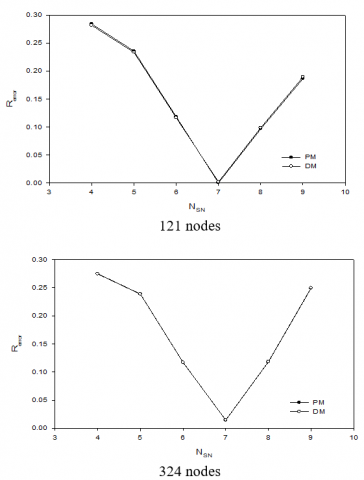Figure 2. Variation of relative error for NSN by taking PM and DM for the enforcement of EBC

## 3.pngFigure 3. Variation of relative error for NSB by taking PM and DM for the enforcement of EBC

## 4.png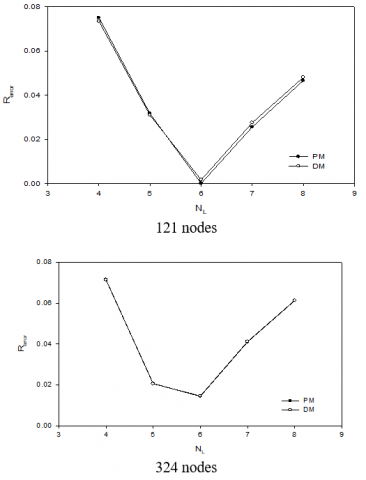Figure 4. Variation of relative error for NL by taking PM and DM for the enforcement of EBC

Table 2. Comparison of MLPG results with analytical solution under steady-state conditions at x= H/2, y= D/2 of the duct cross-section

 S. No. Number of nodes Analytical solution result MLPG results v, m/s % Error PM 1 121 1.1802 1.1801 0.0085 2 324 1.1972 1.4404 DM 1 121 1.1802 1.1822 0.1695 2 324 1.1975 1.4659

Table 2 shows an error estimate for the MLPG method at the centre of the duct cross-section. The maximum error obtained by the PM and DM has been found to be 1.44% and 1.46% respectively at 324 nodes. However, this error is 0.0085% and 0.1695% respectively at 121 nodes; hence, the results obtained by the MLPG method at 121 nodes are more accurate than 324 nodes. Also, the PM is found to be more accurate than DM.

4.2 Transient analysis

The analysis of transient model has been carried out in this section by the MLPG method by using the PM and DM at 121 and 324 nodes respectively. A study to identify the optimized range of parameters have been conducted and explored as m=3, NSN= 7, NSB=7 and NL=6 for 121 nodes and m=3, NSN= 7, NSB=7 and NL=5 for 324 nodes respectively.

## 5.png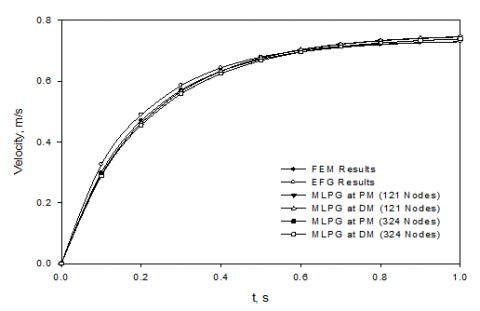Figure 5. Transient two-dimensional analysis of fluid flow problem at x= H/4, y= D/4

## 6.pngFigure 6. Velocity of fluid at x/H = 0.0125 obtained by the MLPG method using PM

## 7.pngFigure 7. Velocity of fluid at x/H = 0.025 obtained by the MLPG method using PM

## 8.png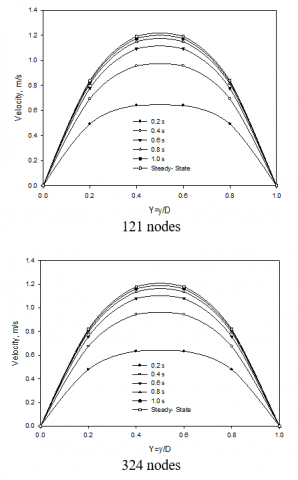Figure 8. Velocity of fluid at x/H = 0.050 obtained by the MLPG method using PM

## 9.png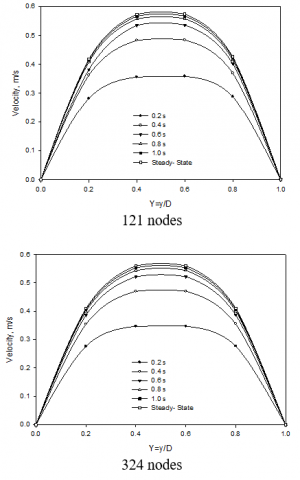Figure 9. Velocity of fluid at x/H = 0.0125 obtained by the MLPG method using DM

## 10.png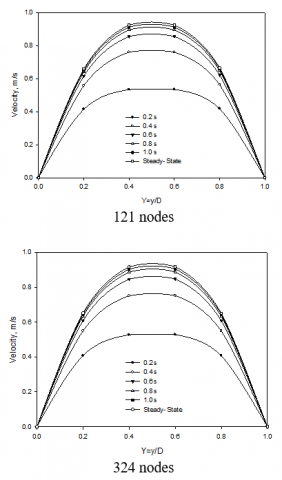Figure 10. Velocity of fluid at x/H = 0.025 obtained by the MLPG method using DM

## 11.png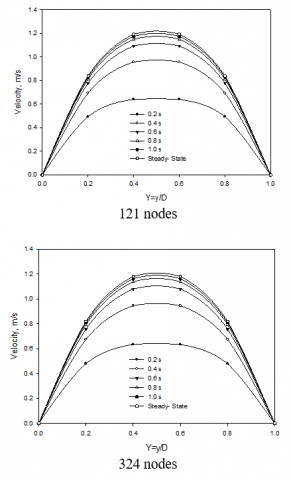Figure 11. Velocity of fluid at x/H = 0.050 obtained by the MLPG method using DM

A comparison of the MLPG results and the benchmark method results  at x= H/4, y= D/4 for 121 and 324 node numbers respectively are demonstrated in Fig. 5. The results obtained by the MLPG results are found to be in good agreement with the benchmark method results; also, the figure demonstrates the superiority of the PM over DM.

Figs. 6-8 show the progression of fluid velocity with time at different locations along the cross-section of the duct till the attainment of steady-state by the MLPG method for 121 and 324 nodes respectively.

The EBC is imposed by the PM. The velocity is found to be least near the walls of the duct and most at the centre of the cross-section i.e at x/H = 0.050.

Figs. 9-11 show the progression of fluid velocity with time at different locations along the cross-section of the duct till the attainment of steady-state by the MLPG method for 121 and 324 nodes respectively. The EBC is imposed by the DM. The velocity is found to be least near the walls of the duct and most at the centre of the cross-section i.e at x/H = 0.050.

It is because of the boundary conditions imposed on the four boundaries of the duct cross-section (refer Eqn. 10). At and near the surfaces the velocity of fluid is assumed to be negligible; however, the fluid velocity apart from the surface gradually increases and reaches to maximum at the centre due to no or less effect of boundary conditions. It can also be observed that after certain period of time the time-profiles attain the steady-state.

5. Conclusions

The MLPG method, due to its flexibility in formulation is employed to investigate the fluid flow problems. Careful selection of parameters yield the accurate results. In the steady-state and transient two-dimensional fluid flow problem the EBC is imposed both by the DM and PM respectively. Two-level q method has been employed in both the problems for temporal discretization. The fourth order spline weight function has been used to interpolate the test function. C++ codes have been developed to model the sample problems. The results obtained by the model problems have been compared with that of the analytical and benchmark solutions and found to be in very good agreement. The fluid velocity is found to be highest at the centre of the cross-section of the duct. Finally, it can be concluded that the MLPG method can be a good choice to solve the fluid flow though a long and uniform duct with meshing and remeshing of computational domain.

Nomenclature
 A Cross-sectional area of duct, m2 a(x) Vector of coefficients A(x) Weighted momentum matrix D Bredth of duct, m F Load vector H Length of duct, m K Stiffness matrix p(x) Basis function ∆t Time step, s uf Fluid velocity, m/s us Fluid velocity along the surface of duct, m/s ui Nodal parameter of u at x=xi uini Initial velocity of fluid, m/s u(x) Unknown scalar function of a field variable v Test function for MLPG method w Weight function wi Gauss weights x Coordinate in x- direction y Coordinate in y- direction Greek symbols a Penalty parameter b thermal expansion coefficient, K-1 Γ Boundary of global domain Γ1 Boundary of the top surface of the duct Γ2 Boundary of the left surface of the duct Γ3 Boundary of the bottom surface of the duct Γ3 Boundary of the right surface of the duct μ Dynamic viscosity, Ns/m2 ρ Density of material, kg/m3 Φ MLS shape function Ω Domain ΩQ Local domain ∂ΩQ Boundary of local domain
References

 Lucy L. (1977). A numerical approach to testing the fission hypothesis. Astron J. 82: 1013-1024.

 Gingold RA, Moraghan JJ. (1977). Smoothed particle hydrodynamics: Theory and applications to non spherical stars. Man. Not. Roy., Astron. Soc. 181: 375-389.

 Liu GR, Liu MB. (2003). Smoothed Particle hydrodynamics-A meshfree particle method. World Scientific, Singapore.

 Nyroles B, Touzot G, Villon P. (1992). Generalizing the finite element method: Diffuse approximation and diffuse elements. Computational Mechanics 10: 307-318.

 Zhu T, Zhang JD, Atluri SN. (1998). A local boundary integral equation (LBIE) method in computational mechanics, and a meshless discretization approach. Comput. Mech. 21: 223-235.

 Sladek J, Sladek V, Atluri SN. (2002). Application of the local boundary integral equation method to boundary-value problems. Int. Appl. Mech. 38(9): 1025-1047.

 Belytschko T, Lu YY, Gu L. (1994). Element-free Galerkin methods. Int. J. Numer. Methods Engrg. 37: 229-256.

 Singh IV. (2004). Application of meshless EFG method in fluid flow problems. Sadhana 29(3): 285-296.

 Onate E, Idelsohn S, Zienkiewicz OC, Taylor RL, Sacco C. (1996). A stabilized finite point method for analysis of fluid mechanics problems. Comput. Methods Appl. Mech. Engrg. 139: 315-346.

 Atluri SN, Zhu T. (1998a). A new meshless local Petrov-Galerkin (MLPG) approach to nonlinear problems in computer modeling and simulation. CMES 3(3): 187-196.

 Atluri SN, Zhu T. (1998b). A new meshless local Petrov-Galerkin (MLPG) approach in computational mechanics. Computational Mechanics 22: 117-127.

 Atluri SN, Shen S. (2005). The basis of meshless domain discretization: the meshless local Petrov-Galerkin (MLPG) method. Advances in Computational Mathematics 23: 73-93. https://doi.org/10.1007/s10444-004-1813-9

 Liu GR, Gu YT. (2001a). A point interpolation method for two-dimensional solids. Int. J. Muner. Meth. Engng. 50: 937-951.

 Liu GR, Gu, YT. (2001b). A local radial point interpolation method (LR-PIM) for free vibration analysis of 2-D solids. J. of Sound and Vibration 246(1): 29-46.

 Wang JG, Liu GR. (2000). Radial point interpolation method for elastoplastic problems. Proc. of the 1st Int. Conf. on Structural Stability and Dynamics, Dec.7-9, 2000, Taipei, Taiwan, 703-708.

 Wang JG, Liu GR. (2002). A point interpolation meshless method based on radial basis functions. Int. J. Numer. Meth. Eng. 54(11): 1623-1648. https://doi.org/10.1002/nme.489

 Liu GR, Gu YT. (1996). A meshfree method: Meshfree Weak-Strong (MWS) form method, for 2-D Solids. Comput. Mech. 33(1): 2-14.

 Garg R, Thakur H, Tripathi B. (2015). A review of applications of meshfree methods in the area of heat transfer and fluid flow: MLPG method in particular. International Research Journal of Engineering and Technology 2(4): 329-338.

 Garg R, Thakur H, Tripathi B. (2017a). Nonlinear and transient heat transfer in the fin by a truly meshless method. Indian Journal of Science and Technology 10(31). https://doi.org/10.17485/ijst/2017/v10i31/113859

 Garg R, Thakur H, Tripathi B. (2017b). Nonlinear numerical analysis of convective-radiative fin using MLPG method. International Journal of Heat and Technology 35(4): 721-729. https://doi.org/10.18280/ijht.350405

 Thakur HC, Singh KM, Sahoo PK. (2010). Meshless local Petrov-Galerkin method for nonlinear heat conduction problems. Numer. Heat Transfer B, Part B 56: 393- 410. https://doi.org/10.1080/10407790903508152

 Thakur HC, Singh KM, Sahoo PK. (2011). MLPG analysis of nonlinear heat conduction in irregular domains. CMES 68 (2): 117-149. https://doi.org/10.3970/cmes.2010.068.117

 Thakur HC. (2010). Meshless Local Petrov-Galerkin Method for Phase Change Problems. Ph.D Dissertation. Department of Mechanical Engineering, IIT Roorkee.

 Lin H, Atluri SN. (2001). The Meshless Local Petrov-Galerkin method for solving incompressible Navier- Stokes equations. CMES 2(2): 117-142.

 Wu YL, Liu GR, Gu YT. (2005). Application of meshless local Petrov-Galerkin (MLPG) approach to simulation of incompressible flow. Numer. Heat Tran. B. 48: 459-475. https://doi.org/10.1080/10407790500324763

 Avila R, Atluri S N. (2009). Numerical solution of non-steady flows, around surfaces in spatially and temporally arbitrary motions, by using the MLPG method. CMES 54(1): 15-64. https://doi.org/10.3970/cmes.2009.054.015

 Wu XH, Tao WQ, Shen SP, Zhu XW. (2010). A stabilized MLPG method for steady state incompressible fluid flow simulation. Journal of Computational Physics 229(22): 8564-8577. https://doi.org/10.1016/j.jcp.2010.08.001

 Avila R, Han Z, Atluri SN. (2011). A novel MLPG-Finite-Volume mixed method for analyzing Stokesian flows and study of a new vortex mixing flow. CMES 71(4): 363-396. https://doi.org/10.3970/cmes.2011.071.363

 Arefmanesh A, Tavakoli, M. (2012). Nanofluid natural convection in a 3D cubic cavity using the Meshless Local Petrov-Galerkin method, Proc. of the 4th Intl. Conf. on Nanostructures (ICNS4) 12-14 March, 2012, Kish Island, I.R. Iran.

 Sataprahm C, Luadsong A. (2013). The meshless local Petrov-Galerkin method for simulating unsteady incompressible fluid flow. J. the Egyptian Mathematical Society 1-10. https://doi.org/10.1016/j.joems.2013.10.002

 Enjilela V, Arefmanesh A. (2015). Two-step Taylor- characteristic-based MLPG method for fluid flow and heat transfer applications, Engineering Analysis with Boundary Elements 51: 174-190. https://doi.org/10.1016/j.enganabound.2014.10.012

 Zhu T, Atluri SN. (1998). A modified collocation method and a penalty formulation for enforcing the essential boundary conditions in the element free Galerkin method. Computational Mechanics (Springer- Verlag) 21: 211-222. https://doi.org/10.1007/s004660050296

 Lancaster P, Salkauskas K. (1981). Surfaces generated by moving least squares methods. Math. Comput. 37: 141-158. https://doi.org/10.1090/S0025-5718-1981-0616367-1

 Liu GR, Gu YT. (2005). An Introduction to Meshfree Methods and their Programming. Springer.

 Wood WL. (1990). Practical Time-stepping Schemes. Oxford Applied Mathematics and Com-puting Science Series Clarendon Press, Oxford. https://doi.org/10.1002/nme.1620310115